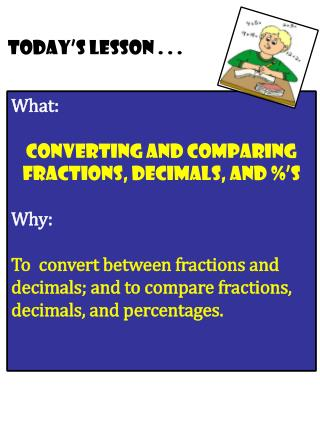DownloadDownload PresentationWhat: converting and Comparing Fractions, Decimals, and %’s Why:

# What: converting and Comparing Fractions, Decimals, and %’s Why:

Download Presentation## What: converting and Comparing Fractions, Decimals, and %’s Why:

- - - - - - - - - - - - - - - - - - - - - - - - - - - E N D - - - - - - - - - - - - - - - - - - - - - - - - - - -
##### Presentation Transcript

1. Today’s lesson . . . What: converting and Comparing Fractions, Decimals, and %’s Why: To convert between fractions and decimals; and to compare fractions, decimals, and percentages.

2. Converting Decimals to Fractions: Think about decimal language! Read it, Write it, Reduce it! Convert the following decimal #’s into fractions. Remember, if you can say the decimal #’s in your head (using decimal language)– you can write the decimal as a fraction! READ IT, WRITE IT, REDUCE IT!

3. Continued: Read it, Write it, Reduce it! How do you change a fraction into a decimal?? Video

4. “Take the top #, divide by the bottom #!” Converting Fractions to Decimals: 5 8 GuIDED PRACTICE:

6. Comparing Fractions, Decimals, and %’s: STRATEGY: Convert everything into decimals first! Let’s practice COMPARING a MIXTURE of fractions, decimals, and %’s at the same time. Remember, CONVERT TO DECIMALS FIRST!!!! Guided practice: Place a “greater-than” (>), “less-than” (<), or “equal” (=) sign in the blanks: 0.023 _____ 0.6 2) 35% _____ 3.5 3) 2/5 _____ 40% 4) 2.5% _____ ¼ 5) 0.5 _____ 5% 6) 0.75% _____ 0.75

7. Your turn: Place a “greater-than” (>),“less-than” (<), or “equal” (=) sign in the blanks: 4.5 _____ 45% 2) 15% _____ 0.092 3) 3/8 _____ 0.4 4) 6.8% _____ 0.68 5) 20% _____ 1/5 6) 5.09 _____ 450%

8. END OF LESSON Next slides are student copies of the notes for this lesson. These notes were handed out in class and filled-in as the lesson progressed. NOTE: The last slides in any lesson slideshow (entitled “Practice Work”) represent the homework assigned for that day.

9. NOTES DATE: ______/_______/_______ NAME: What: converting and Comparing Fractions, Decimals, and %’s Why: To convert between fractions and decimals, and to compare fractions, decimals, and percentages. Converting Decimals to Fractions: Think about decimal language! Read it, Write it, Reduce it! EXAMPLES: Convert the following decimal #’s into fractions. Remember, if you can say the decimal #’s in your head (using decimal language)– you can write the decimal as a fraction! READ IT, WRITE IT, REDUCE IT!

10. “Take the top #, divide by the bottom #!” Converting Fractions to Decimals: GuIDED PRACTICE: Convert the following fractions into decimals. 5 8 Your Turn: